Courses

# NCERT Exemplar Solutions: Number System Class 6 Notes | EduRev

## Class 6: NCERT Exemplar Solutions: Number System Class 6 Notes | EduRev

The document NCERT Exemplar Solutions: Number System Class 6 Notes | EduRev is a part of the Class 6 Course Mathematics (Maths) Class 6.
All you need of Class 6 at this link: Class 6

Exercise Page: 5

In questions 1 to 38, out of the four options, only one is correct. Write the correct answer.
Q.1. The product of the place values of two 2’s in 428721 is
(a) 4
(b) 40000
(c) 400000
(d) 40000000
Ans: (c)
Explanation:
The product of the place values of two 2’s in 428721 is
There are two 2’s in the given number.
So, the first 2 is in the tenth place,
Then, the product is = 2 × 10
= 20
The other 2 is in place value of ten thousand.
Then, = 2 × 10000
= 20000
Therefore, the product of place values = 20 × 20000
= 400000

Q.2. 3 × 10000 + 7 × 1000 + 9 × 100 + 0 ×10 + 4 is the same as
(a) 3794
(b) 37940
(c) 37904
(d) 379409
Ans: (c)
Explanation:
3 × 10000 = 30000
The place value is 30000
7 × 1000 = 7000
The place value is 7000
9 × 100 = 900
The place value is 900
0 × 10 = 0
4 = 4
Therefore, the sum of all place value is = 30000 + 7000 + 900 + 0 + 4
= 37904

Q.3. If 1 is added to the greatest 7- digit number, it will be equal to
(a) 10 thousand
(b) 1 lakh
(c) 10 lakh
(d) 1 crore
Ans: (d)
Explanation:
We know that, the greatest number is 99,99,999
Then, 1 is added to 99,99,999 = 99,99,999 + 1
= 1,00,00,000
= 1 crore

Q.4. The expanded form of the number 9578 is
(a) 9 × 10000 + 5 × 1000 + 7 × 10 + 8 × 1
(b) 9 × 1000 + 5 × 100 + 7 × 10 + 8 × 1
(c) 9 × 1000 + 57 × 10 + 8 × 1
(d) 9 × 100 + 5 × 100 + 7 × 10 + 8 × 1
Ans: (b)
Explanation:
Consider the given number 9578,
The place value of 8 is ones = 8 × 1
The place value of 7 is tens = 7 × 10
The place value of 5 is thousand = 5 × 100
The place value of 9 is ten thousand = 9 × 1000

Q.5. When rounded off to nearest thousands, the number 85642 is
(a) 85600
(b) 85700
(c) 85000
(d) 86000
Ans: (d)
Explanation: When rounded off to nearest thousands, the number 85642 is = 86000

Q.6. The largest 4-digit number, using any one digit twice, from digits 5, 9, 2 and 6 is
(a) 9652
(b) 9562
(c) 9659
(d) 9965
Ans: (d)
Explanation: Using 9 as twice from 5, 9, 2 and 6, then then number is 9965.

Q.7. In Indian System of Numeration, the number 58695376 is written as
(a) 58,69, 53, 76
(b) 58,695,376
(c) 5,86,95,376
(d) 586,95,376
Ans: (c)
Explanation: In Indian System of Numeration, the number 58695376 is written as 5 crore, eighty six lakh, ninety five thousand, three hundred and seventy six = 5,86,95,376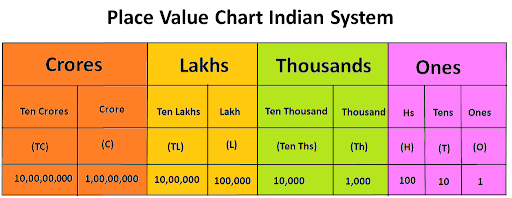India Place Value Chart

Q.8. One million is equal to
(a) 1 lakh
(b) 10 lakh
(c) 1 crore
(d) 10 crore
Ans: (b)
Explanation:
One million is equal to ten lakh.
1,000,000 = 10,00,000

Q.9. The greatest number which on rounding off to nearest thousands gives 5000, is
(a) 5001
(b) 5559
(c) 5999
(d) 5499
Ans: (d)
Explanation: The greatest number which on rounding off to nearest thousands gives 5000, is 5499.

Q.10. Keeping the place of 6 in the number 6350947 same, the smallest number obtained by rearranging other digits is
(a) 6975430
(b) 6043579
(c) 6034579
(d) 6034759
Ans: (c)
Explanation: Keeping the place of 6 in the number 6350947 same, the smallest number obtained by rearranging other digits is 6034579.

Q.11. Which of the following numbers in Roman numerals is incorrect?
(a) LXXX
(b) LXX
(c) LX
(d) LLX
Ans: (d)
Explanation: As we know that, the symbol L can never be repeated.
Therefore, LLX is incorrect.

Q.12. The largest 5-digit number having three different digits is
(a) 98978
(b) 99897
(c) 99987
(d) 98799
Ans: (c)
Explanation: In the given, options there are three numbers used 9, 8 and 7
To get the largest of 5- digit we have to arrange the numbers in descending order.
Then, from the given options 99987 is the largest of 5 – digit number.

Q.13. The smallest 4-digit number having three different digits is
(a) 1102
(b) 1012
(c) 1020
(d) 1002
Ans: (d)
Explanation: In the given, options there are three numbers used 0, 1 and 2

To get the largest of 4- digit we have to arrange the numbers in ascending order.

Then, from the given options 1002 is the smallest of 4 – digit number.

Q.14. Number of whole numbers between 38 and 68 is
(a) 31
(b) 30
(c) 29
(d) 28
Ans: (c)
Explanation: Number of whole numbers between 38 and 68 is 29.

Q.15. The product of successor and predecessor of 999 is
(a) 999000
(b) 998000
(c) 989000
(d) 1998
Ans: (b)
ExplanationThe number which comes immediately before a particular number is called its predecessor.
The successor of a whole number is the number obtained by adding 1 to it.
So, Successor of 999 = 999 + 1 = 1000
Predecessor = 999 – 1 = 998
Then, product of successor and predecessor of 999 is = 1000 × 998
= 998000

Q.16. The product of a non-zero whole number and its successor is always
(a) an even number
(b) an odd number
(c) a prime number
(d) divisible by 3
Ans: (a)
ExplanationThe product of a non-zero whole number and its successor is always an even number.
For example: – 4 × 5 = 20, 7 × 8 = 56

Q.17. A whole number is added to 25 and the same number is subtracted from 25. The sum of the resulting numbers is
(a) 0
(b) 25
(c) 50
(d) 75
Ans: (c)
Explanation
Let us assume the number be x.
From the question it is given that, number is added to 25 = x + 25
The same number is subtracted to from 25 = 25 – x
Then, the sum of the resulting numbers is = (x + 25) + (25 – x)
= x + 25 + 25 – x
= 50 + x – x
= 50 + 0
= 50

Q.18. Which of the following is not true?
(a) (7 + 8) + 9 = 7 + (8 + 9)
(b) (7 × 8) × 9 = 7 × (8 × 9)
(c) 7 + 8 × 9 = (7 + 8) × (7 + 9)
(d) 7 × (8 + 9) = (7 × 8) + (7 × 9)
Ans: (c)
Explanation:
Consider the left hand side = 7 + 8 × 9
= 7 + (8 × 9)
= 7 + 72
= 79
Now, consider the right hand side = (7 + 8) × (7 + 9)
= 15 × 16
= 240
By comparing LHS and RHS
LHS ≠ RHS
79 ≠ 240

Q.19. By using dot (.) patterns, which of the following numbers can be arranged in all the three ways namely a line, a triangle and a rectangle?
(a) 9
(b) 10
(c) 11
(d) 12
Ans: (b)
Explanation: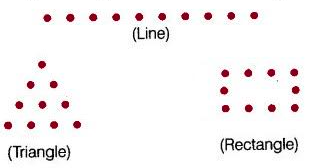Q.20. Which of the following statements is not true?
(a) Both addition and multiplication are associative for whole numbers.
(b) Zero is the identity for multiplication of whole numbers.
(c) Addition and multiplication both are commutative for whole numbers.
(d) Multiplication is distributive over addition for whole numbers.
Ans: (b)

Q.21. Which of the following statements is not true?
(a) 0 + 0 = 0
(b) 0 – 0 = 0
(c) 0 × 0 = 0
(d) 0 ÷ 0 = 0
Ans: (d)
Explanation: Zero divided by zero is not defined.

Q.22. The predecessor of 1 lakh is
(a) 99000
(b) 99999
(c) 999999
(d) 100001
Ans: (b)
Explanation:
The number which comes immediately before a particular number is called its predecessor.
The predecessor of 1 lakh is = 1,00,000 – 1
= 99,999

Q.23. The successor of 1 million is
(a) 2 millions
(b) 1000001
(c) 100001
(d) 10001
Ans: (b)
Explanation:
The successor of a whole number is the number obtained by adding 1 to it.
We know that, 1 million = 10,00,000
Then, successor = 10,00,000 + 1
= 10,00,001

Q.24. Number of even numbers between 58 and 80 is
(a) 10
(b) 11
(c) 12
(d) 13
Ans: (a)
Explanation: Even numbers between 58 and 80 are 60, 62, 64, 66, 68, 70, 72, 74, 76, 78.

Q.25. Sum of the number of primes between 16 to 80 and 90 to 100 is
(a) 20
(b) 18
(c) 17
(d) 16
Ans: (c)
Explanation
Prime numbers between 16 to 80 = 17, 19, 23, 29, 31, 37, 41, 43, 47, 53, 59, 61, 67, 71, 73 and 79.
Then, number of primes between 16 to 80 = 16
Prime numbers between 90 to 100 = 97
Then, number of primes between 90 to 100 = 1
Therefore, Sum of the number of primes between 16 to 80 and 90 to 100 is,
= 16 + 1
= 17

Q.26. Which of the following statements is not true?
(a) The HCF of two distinct prime numbers is 1
(b) The HCF of two co prime numbers is 1
(c) The HCF of two consecutive even numbers is 2
(d) The HCF of an even and an odd number is even.
Ans: (d)
Explanation: The HCF of an even and an odd number is odd number.

Q.27. The number of distinct prime factors of the largest 4-digit number is
(a) 2
(b) 3
(c) 5
(d) 11
Ans: (b)
Explanation:
The largest 4 – digit number = 9999
Prime factors of 9999 = 3 × 3 × 11 × 101
So, 9999 = 32 × 11 × 101
Therefore, distinct prime factors are = 3, 11 and 101
Number of distinct prime factors of the largest 4-digit number is = 3

Q.28. The number of distinct prime factors of the smallest 5-digit number is
(a) 2
(b) 4
(c) 6
(d) 8
Ans: (a)
Explanation:
The smallest 5 – digit number = 10000
Prime factors of 10000 = 2 × 2 × 2 × 2 × 5 × 5 × 5 × 5
So, 10000 = 24 × 54
Therefore, distinct prime factors are = 2 and 5
Number of distinct prime factors of the smallest 5-digit number is = 2

Q.29. If the number 7254*98 is divisible by 22, the digit at * is
(a) 1
(b) 2
(c) 6
(d) 0
Ans: (c)
Explanation:
Take the alternating sum of the digits in the number, read from left to right. If that is divisible by 11, then the original number.
7 – 2 + 5 – 4 + * – 9 + 8 = (5 + *)
For the given number 7254 * 98 to be divisible by 11,
(5 + *) must also be divisible by 11
So, 5 + * = 11
Therefore * = 11 – 5
= 6

Q.30. The largest number which always divides the sum of any pair of consecutive odd numbers is
(a) 2
(b) 4
(c) 6
(d) 8
Ans: (b)
Explanation:
1 + 3 = 4 = 4/4 = 1
3 + 5 = 8 = 8/4 = 2
The largest number which always divides the sum of any pair of consecutive odd numbers is 4.

Q.31. A number is divisible by 5 and 6. It may not be divisible by
(a) 10
(b) 15
(c) 30
(d) 60
Ans: (d)
Explanation:
The LCM of 6 and 5 is 30.
So, 30 is divisible by 10, 15 and 30 in the given options.
But, 30 is not divisible by 60.

Q.32. The sum of the prime factors of 1729 is
(a) 13
(b) 19
(c) 32
(d) 39
Ans: (d)
Explanation:
The prime factors of 1729 = 7 × 13 × 19
Therefore, the sum of prime numbers = 7 + 13 + 19
= 39

Q.33. The greatest number which always divides the product of the predecessor and successor of an odd natural number other than 1, is
(a) 6
(b) 4
(c) 16
(d) 8
Ans: (b)
Explanation:
Let us assume an odd natural number be 5.
Then, predecessor of 5 = 5 -1 = 4
Successor of 5 = 5 + 1 = 6
Then, the product of predecessor and successor = 4 × 6
= 24
24 is divided by 4 = 24/4 = 6
Therefore, the greatest number which always divides the product of the predecessor and successor of an odd natural number other than 1, is 4.

Q.34. The number of common prime factors of 75, 60, 105 is
(a) 2
(b) 3
(c) 4
(d) 5
Ans: (a)
Explanation:
Prime factors of,
75 = 3 × 5 × 5
60 = 2 × 2 × 3 × 5
105 = 3 × 5 × 7
So, common prime factors in the given three numbers are 3 and 5.
Therefore, the number of common prime factors of 75, 60, 105 is 2.

Q.35. Which of the following pairs is not coprime?
(a) 8, 10
(b) 11, 12
(c) 1, 3
(d) 31, 33
Ans: (a)
Explanation:
First of all, both the numbers are even.
Then, common factor of both numbers is 2 other than 1.
Therefore, 8 and 10 are not coprime.

Q.36. Which of the following numbers is divisible by 11?
(a) 1011011
(b) 1111111
(c) 22222222
(d) 3333333
Ans: (c)
Explanation:
To check the divisibility of a number by 11, the rule is, find the difference between the sum of the digits at odd places (from the right) and the sum of the digits at even places (from the right) of the number. If the difference is either 0 or divisible by 11, then the number is divisible by 11.
So, 2 – 2 + 2 – 2 + 2 – 2 + 2 – 2 = 0
Therefore, 22222222 is divisible by 11.

Q.37. LCM of 10, 15 and 20 is
(a) 30
(b) 60
(c) 90
(d) 180
Ans:
(b)
Explanation:
Factors of 10, 15 and 30 is,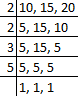Then, LCM of 10, 15 and 30 is 2 × 2 × 3 × 5 × 1 = 60

Q.38. LCM of two numbers is 180. Then which of the following is not the HCF of the numbers?
(a) 45
(b) 60
(c) 75
(d) 90
Ans:
(c)
Explanation:
Factors of 180.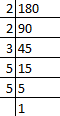Then, factors of 180 = 2 × 2 × 3 × 3 × 5
180 is not divided by 180.
Therefore, 75 is not the HCF of the number 180.

In questions 39 to 98 state whether the given statements are true (T) or false (F).
Q.39. In Roman numeration, a symbol is not repeated more than three times.
Solution: True.
As per the rule of the Roman numerals, a symbol is not repeated more than three times.

Q.40. In Roman numeration, if a symbol is repeated, its value is multiplied as many times as it occurs.
Solution: False.
If a symbol is repeated, its value is added as many times as it occurs: i.e. II is equal 2, XX is 20 and XXX is 30.

Q.41. 5555 = 5 × 1000 + 5 × 100 + 5 × 10 + 5 × 1
Solution: True.
Left Hand Side = 5555
Right Hand Side = 5 × 1000 + 5 × 100 + 5 × 10 + 5 × 1
= 5000 + 500 + 50 + 5
= 5555
Left Hand Side = Right Hand Side

Q.42. 39746 = 3 × 10000 + 9 × 1000 + 7 × 100 + 4 × 10 + 6
Solution: True.
Left Hand Side = 39746
Right Hand Side = 3 × 10000 + 9 × 1000 + 7 × 100 + 4 × 10 + 6
= 30000 + 9000 + 700 + 40 + 6
= 39746
Left Hand Side = Right Hand Side

Q.43. 82546 = 8 × 1000 + 2 × 1000 + 5 × 100 + 4 × 10 + 6
Solution: False.
Left Hand Side = 82546
Right Hand Side = 8 × 1000 + 2 × 1000 + 5 × 100 + 4 × 10 + 6
= 8000 + 2000 + 500 + 40 + 6
= 10,546
Left Hand Side ≠ Right Hand Side

Q.44. 532235 = 5 × 100000 + 3 × 10000 + 2 × 1000 + 2 × 100 + 3 × 10 + 5
Solution: True.
Left Hand Side = 532235
Right Hand Side = 5 × 100000 + 3 × 10000 + 2 × 1000 + 2 × 100 + 3 × 10 + 5
= 5,00,000 + 30,000 + 2000 + 200 + 30 + 5
= 5,32,235
Left Hand Side = Right Hand Side

Q.45. XXIX = 31
Solution: False.
Where, X = 10
IX = 9
So, XXIX = 10 + 10 + 9
= 29

Q.46. LXXIV = 74
Solution: True.
Where, L = 50
X = 10
IV = 4
So, LXXIV = 50 + 10 + 10 + 4
= 74

Q.47. The number LIV is greater than LVI.
Solution: False.
Where, L = 50
IV = 4
VI = 6
So, LIV = 50 + 4 = 54
LVI = 50 + 6
Therefore, 54 < 56
Hence, LIV < LVI

Q.48. The numbers 4578, 4587, 5478, 5487 are in descending order.
Solution: False.
In the question, the arrangement of the numbers in ascending order.
Descending order of the given number = 5487, 5478, 4587, 4578.

Q.49. The number 85764 rounded off to nearest hundreds is written as 85700.
Solution: False.
The number 85764 rounded off to nearest hundreds is written as 85800.

Q.50. Estimated sum of 7826 and 12469 rounded off to hundreds is 20,000.
Solution: True.
The number 7826 rounded off to nearest hundreds is written as 7800.
The number 12469 rounded off to nearest hundreds is written as 12500
So, sum of numbers after rounded off to hundreds = 7800 + 12500 = 20,300
Therefore, 20,300 is nearest to 20,000.

Q.51. The largest six digit telephone number that can be formed by using digits 5, 3, 4, 7, 0, 8 only once is 875403.
Solution: False.
The largest six digit telephone number that can be formed by using digits 5, 3, 4, 7, 0, 8 only once is 875430.

Q.52. The number 81652318 will be read as eighty one crore six lakh fifty two thousand three hundred eighteen.
Solution: False.
The given number 8,16,52,318 will be read as eight crore sixteen lakh fifty two thousand three hundred and eighteen.

Q.53. The largest 4-digit number formed by the digits 6, 7, 0, 9 using each digit only once is 9760.
Solution: True.

Q.54. Among kilo, milli and centi, the smallest is centi.
Solution: False.
Among kilo, milli and centi, the smallest is milli.

Q.55. Successor of a one-digit number is always a one-digit number.
Solution: False.
The successor of a whole number is the number obtained by adding 1 to it.
Example: – consider the number 9 it is a one digit, then its successor = 9 + 1 = 10.

Q.56. Successor of a 3-digit number is always a 3-digit number.
Solution: False.
The successor of a whole number is the number obtained by adding 1 to it.
Example: – consider 3-digit number 999 it is a one digit, then its successor = 999 + 1 = 1000.

Q.57. Predecessor of a two-digit number is always a two-digit number.
Solution: False.
The number which comes immediately before a particular number is called its predecessor.
Example: – consider 2-digit number 10, then its predecessor = 10 – 1 = 9.

Q.58. Every whole number has its successor.
Solution: True.

Q.59. Every whole number has its predecessor.
Solution: False.
Consider the whole number 0,
Then, its predecessor = 0 – 1 = -1
– 1 is an integer.

Q.60. Between any two natural numbers, there is one natural number.
Solution: False.
Consider the two natural numbers 4 and 8.
Then, natural numbers between 4 and 8 are 5, 6, 7.

Q.61. The smallest 4-digit number is the successor of the largest 3-digit number.
Solution: True.
The successor of a whole number is the number obtained by adding 1 to it.
The largest 3-digit number = 999
Then, its successor = 999 + 1
= 1000

Q.62. Of the given two natural numbers, the one having more digits is greater.
Solution: True.
As per the rule, Of the given two natural numbers, the one having more digits is greater.

Q.63. Natural numbers are closed under addition.
Solution: True.
We know that, sum of two natural numbers is always natural number.
Therefore, natural numbers are closed under addition.

Q.64. Natural numbers are not closed under multiplication.
Solution: False.
We know that, multiplication of two natural numbers is always natural number.
Therefore, natural numbers are closed under multiplication.

Q.65. Natural numbers are closed under subtraction.
Solution: False.
Difference of two natural numbers are not always a natural number.
Therefore, natural numbers are not closed under subtraction.

Q.66. Addition is commutative for natural numbers.
Solution: True.
Let us assume ‘a’ and ‘b’ are the two natural numbers.
Then commutative for natural numbers is a + b = b + a.
Consider the two natural numbers 2 and 4.
Where, a = 2, b = 4
a + b = b + a
2 + 4 = 4 + 2
6 = 6

Q.67. 1 is the identity for addition of whole numbers.
Solution: False.
Zero (0) is the identity for addition of whole numbers.
Consider any whole number i.e. 8.
Then, 8 + 0 = 8

Q.68. 1 is the identity for multiplication of whole numbers.
Solution: True.
Consider any whole number i.e. 6.
6 × 1 = 6

Q.69. There is a whole number which when added to a whole number, gives the number itself.
Solution: True.
Zero (0) is a whole number which when added to a whole number, gives the number itself.

Q.70. There is a natural number which when added to a natural number, gives the number itself.
Solution: False.
We know that, ‘0’ is not a natural number.
Therefore, there is no any natural number which when added to a natural number, gives the number itself.

Q.71. If a whole number is divided by another whole number, which is greater than the first one, the quotient is not equal to zero.
Solution: True.
As per the standard rule, if a whole number is divided by another whole number, which is greater than the first one, the quotient is not equal to zero.

Q.72. Any non-zero whole number divided by itself gives the quotient 1.
Solution: True.
Consider any non-zero whole number i.e. 5
5 is divided by itself = 5/5 = 1

Q.73. The product of two whole numbers need not be a whole number.
Solution: False.
The product of two whole number is always a whole number.
Because, we know that, whole numbers are closed under multiplication.

Q.74. A whole number divided by another whole number greater than 1 never gives the quotient equal to the former.
Solution: True.
As per the standard rule, a whole number divided by another whole number greater than 1 never gives the quotient equal to the former.

Q.75. Every multiple of a number is greater than or equal to the number.
Solution: True.
As per the standard rule, every multiple of a number is greater than or equal to the number.
2 × 1 = 2
2 × 3 = 6

Q.76. The number of multiples of a given number is finite.
Solution: False.
The number of multiples of a given number is infinite.
Because, we know that numbers are infinite.

Q.77. Every number is a multiple of itself.
Solution: True.
We know that, 1 is the identity for multiplication of whole numbers
Therefore, any number is multiplied by 1 we get the number itself.
Hence, every number is a multiple of itself.

Q.78. Sum of two consecutive odd numbers is always divisible by 4.
Solution: True.
For example, 1 + 3 = 4 = 4/4 = 1
11 + 13 = 24 = 24/4 = 6

Q.79. If a number divides three numbers exactly, it must divide their sum exactly.
Solution: True.
As per the standard rule, if a number divides three numbers exactly, it must divide their sum exactly.
Let us consider one number i.e. 2, it divides 4, 6 and 8.
Then, sum of three numbers = 4 + 6 + 8
= 18 is exactly divisible by 2

Q.80. If a number exactly divides the sum of three numbers, it must exactly divide the numbers separately.
Solution: False.

Q.81. If a number is divisible both by 2 and 3, then it is divisible by 12.
Solution: False.
Let us consider the number 6, it is actually divisible by 2 and 3, But 6 is not divisible by 12.

Q.82. A number with three or more digits is divisible by 6, if the number formed by its last two digits (i.e., ones and tens) is divisible by 6.
Solution: False.

Q.83. A number with 4 or more digits is divisible by 8, if the number formed by the last three digits is divisible by 8.
Solution: True.
As per the rule of divisibility test, a number with 4 or more digits is divisible by 8, if the number formed by the last three digits is divisible by 8.

Q.84. If the sum of the digits of a number is divisible by 3, then the number itself is divisible by 9.
Solution: False.
As per the rule of divisibility test, the sum of the digits of a number is divisible by 9, then the number itself is divisible by 9.

Q.85. All numbers which are divisible by 4 may not be divisible by 8.
Solution: True.
Consider the number 20, it is divisible by 4 but not divisible by 8.

Q.86. The Highest Common Factor of two or more numbers is greater than their Lowest Common Multiple.
Solution: False.
The Highest Common Factor of two or more numbers is lower than their Lowest Common Multiple.

Q.87. LCM of two or more numbers is divisible by their HCF.
Solution: True.
As per the rule, LCM of two or more numbers is divisible by their HCF.

Q.88. LCM of two numbers is 28 and their HCF is 8.
Solution: False.
From the question it is given that, LCM of two numbers is 28 and their HCF is 8.
But, 28 is not exactly divide by 8.

Q.89. LCM of two or more numbers may be one of the numbers.
Solution: True.
Consider the two numbers 2 and 4.
Then, LCM of 2 and 4 is 4.

Q.90. HCF of two or more numbers may be one of the numbers.
Solution: True.

Q.91. Every whole number is the successor of another whole number.
Solution: False.
We know that, 0 is the whole number.
0 is no the successor of another whole number.

Q.92. Sum of two whole numbers is always less than their product.
Solution: False.
For example:-
2 + 3 = 5
2 × 3 = 6
From the above example, we can say that sum of two whole numbers is not always less than their product.

Q.93. If the sum of two distinct whole numbers is odd, then their difference also must be odd.
Solution: True.
Consider the two odd numbers 2 and 5.
Then, sum = 2 + 5 = 7 it is an odd number.
Now, difference = 2 – 5 = 3 it also an odd number.

Q.94. Any two consecutive numbers are coprime.
Solution: True.
Co-prime number is a set of numbers or integers which have only 1 as their common factor i.e. their highest common factor (HCF) will be 1. Co-prime numbers are also known as relatively prime or mutually prime numbers. It is important that there should be two numbers in order to form co-primes.

Q.95. If the HCF of two numbers is one of the numbers, then their LCM is the other number.
Solution: True.

Q.96. The HCF of two numbers is smaller than the smaller of the numbers.
Solution: False.
The HCF of two numbers is either greater than or equal to the smaller of the numbers.

Q.97. The LCM of two numbers is greater than the larger of the numbers.
Solution: False.
The LCM of two numbers may be equal to or greater than the larger of the numbers.

Q.98. The LCM of two coprime numbers is equal to the product of the numbers.
Solution: True.

In questions 99 to 100, fill in the blanks to make the statements true.
Q.99. (a) 10 million = _____ crore.
Solution:
10 million = 1 crore
We know that, 1 million = 10 lakh
Then, 10 million = 10 × 10 = 100 lakh = 1,00,00,000
Therefore, 10 million = 1 crore.

(b) 10 lakh = _____ million.
Solution:
10 lakh = 1 million.

Q.100. (a) 1 metre = _____ millimetres.
Solution:
1 metre = 1000millimetres
We know that, 1 metre = 100 centimetre
1 centimetre = 10 millimeter
Then, 100 cm = 10 × 100 = 1000 millimetres

(b) 1 centimetre = _____ millimetres.
Solution:
1 centimetre = 10 millimetres.

(c) 1 kilometre = _____ millimetres.
Solution:
1 kilometre = 10,00,000 millimetres.
We know that, 1 km = 1000 meters.
1 metre = 100 centimetre
1000 metre = 1000 × 100
= 1,00,000 centimetre
1 cm = 10 millimetres
Then, 1,00,000 centimetre = 10 × 1,00,000 = 10,00,000 millimetres

The document NCERT Exemplar Solutions: Number System Class 6 Notes | EduRev is a part of the Class 6 Course Mathematics (Maths) Class 6.
All you need of Class 6 at this link: Class 6Use Code STAYHOME200 and get INR 200 additional OFF Use Coupon Code
All Tests, Videos & Notes of Class 6: Class 6

### Top Courses for Class 6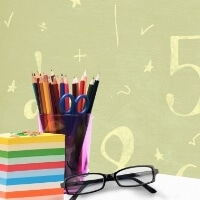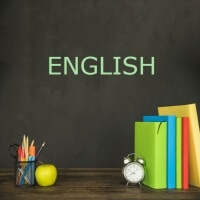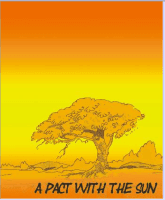## Mathematics (Maths) Class 6

185 videos|229 docs|43 tests

### Top Courses for Class 6Track your progress, build streaks, highlight & save important lessons and more!

,

,

,

,

,

,

,

,

,

,

,

,

,

,

,

,

,

,

,

,

,

;